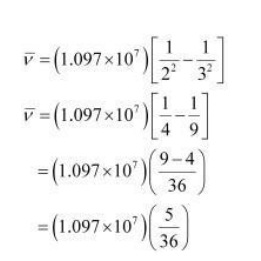# Calculate the wave number for the longest wavelength transitionQuestion.

Calculate the wave number for the longest wavelength transition in the Balmer series of atomic hydrogen.

Solution:

For the Balmer series, $n_{i}=2$. Thus, the expression of wavenumber $(\bar{v})$ is given by,

$\bar{v}=\left[\frac{1}{(2)^{2}}-\frac{1}{n_{\mathrm{f}}^{2}}\right]\left(1.097 \times 10^{7} \mathrm{~m}^{-1}\right)$

Wave number $(\bar{v})$ is inversely proportional to wavelength of transition. Hence, for the

longest wavelength transition, $(\bar{v})$ as to be the smallest.

For $(\bar{v})$ to be minimum, $n_{f}$ should be minimum.

For the Balmer series, a transition from $n_{i}=2$ to $n_{f}=3$ is allowed.

Hence, taking $n_{\mathrm{f}}=3$, we get:$\bar{v}=1.5236 \times 10^{6} \mathrm{~m}^{-1}$